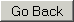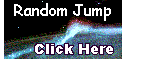Index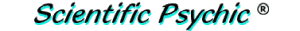#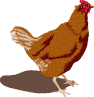The Chicken and Egg Problem

A chicken farmer has figured out that a hen and a half can lay an egg and a half in a day and a half. How many hens does the farmer need to produce one dozen eggs in six days?

This is a classic problem that many people get wrong because they reason that half of a hen cannot lay an egg, and a hen cannot lay half an egg. However, we can get a satisfactory solution by treating this as a purely mathematical problem where the numbers represent averages.

To solve the problem, we first need to find the rate at which the hens lay eggs. The problem can be represented by the following equation, where RATE is the number of eggs produced per hen·day:

1½ hens × 1½ days × RATE = 1½ eggs

We convert this to fractions thus:
3/2 hens × 3/2 days × RATE = 3/2 eggs

Multiplying both sides of the equation by 2/3, we get:
1 hen × 3/2 days × RATE = 1 egg

Multiplying both sides of the equation again by 2/3 and solving for RATE, we get:
RATE = 2/3 eggs per hen·day

Now that we know the rate at which hens lay eggs, we can calculate how many hens (H) can produce 12 eggs in six days using the following equation:

H hens × 6 days × 2/3 eggs per hen·day = 12 eggs

Solving for H, we get:
H = 12 eggs /(6 days × 2/3 eggs per hen·day) = 12/4 = 3 hens

Therefore, the farmer needs 3 hens to produce 12 eggs in 6 days.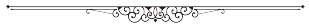Steve Salazar Jr. sent the following comment:

Maybe a little chart will help some of your readers understand "The Chicken and Egg Problem". I also thought you were wrong until I did this little chart.

 Notes hens eggs days 1.5 eggs/1.5 hens/1.5 days 1.5 1.5 1.5 Doubling the hens will double the eggs, but not the days. 3 3 1.5 Then doubling the days will double the eggs, but not the hens. 3 6 3 Double the days again (to reach 6 days) will double the eggs again, but not the hens. 3 12 6Reader Dan sent the following comment:

I think your answer is wrong for The Chicken and Egg Problem. After a load of overcomplex calculations you arrive at the wrong answer of "Therefore, the farmer needs 3 hens to produce 12 eggs in 6 days."

A hen and a half can lay an egg and a half in a day and a half = 1 hen lays 1 egg per day. To simplify it, just double it: 3 hens lay 3 eggs in 3 days = 1 hen lays 1 egg in 1 day.

So 2 hens lay 12 eggs in 6 days.

Unfortunately, Dan has made a mistake by multiplying everything by 2, thereby changing the RATE of egg production. In the original problem, the RATE in eggs per hen·day is:

```              1.5 eggs
RATE = ——————————————————— = 2/3 eggs/hen·day
1.5 hens × 1.5 days

```

By the rules of algebra, you can multiply both the numerator and denominator by 2, which is the same as multiplying by one. So, you can double the number of eggs to 3 in the numerator, and, in the denominator, you can double the hens or double the days, but you cannot double both. By multiplying everything by 2, Dan has really multiplied the RATE by 2/(2×2) or 1/2, changing it from 2/3 to 1/3.Reader Joshua asked:

Regarding the "Chicken and the Egg" problem, I was wondering if you could share with me why Reader Dan's math is false? In your own work of the equation, I have noticed that you neglected to multiply the days of the equation by 2/3 in your second step. Can you please explain to me why you neglected to do so?

Let me address the second question first. When we multiply the equation by 2/3, we have:

2/3×(3/2 hens × 3/2 days × RATE) = 2/3×(3/2 eggs)
1 hen × 3/2 days × RATE = 1 egg

By the commutative laws of algebra, there is no difference between the equation above and:
3/2 hen × days × RATE = 1 eggThe image above may clarify why Reader Dan made a mistake. He multiplied everything times two, doubling the time period, and doubling the number of hens. He interpreted this to mean that three hens will lay three eggs in three days, and therefore that one hen lays one egg per day, which is wrong. The correct postulation of the problem is shown below where a hen and half lays an egg and a half in a day and a half, and by doubling the time period, this same hen and a half lays 3 eggs in 3 days. This gives a rate:

RATE = 3 eggs / (3/2 hen)×3 days = 2/3 eggs per hen·day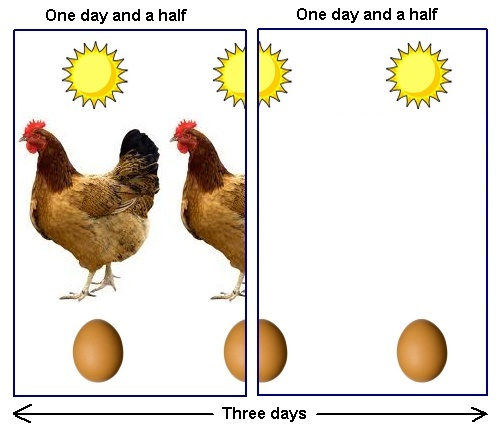Domestication of chickens took place more than 8,000 years ago in what is now Thailand and Vietnam, the region in which red junglefowl are found today. Chicken bones dating to about 7,500 years ago have been found at Neolithic sites at the mouth of the Yellow River in China. Evidence of chicken domestication 4,000 years ago has also been found in Pakistan.

Reference:
Proceedings of the National Academy of Science, v.91, pp. 12505-12509, 12/20/94, Fumihito et al.